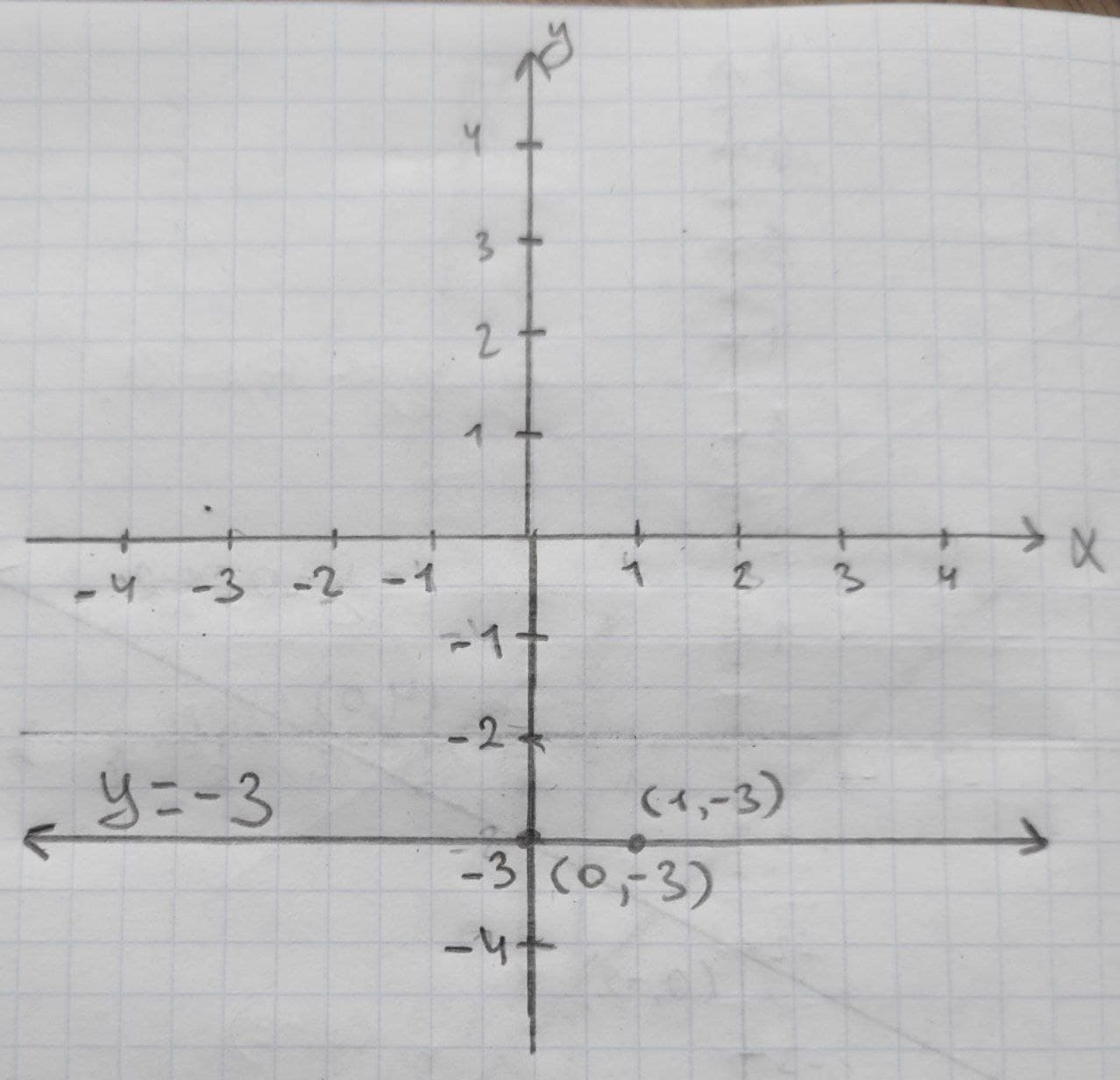# Given linear equation y = -3 a. find the y-intercept and slope. b. determine whether the line slopes upward, slopes downward, or is horizontal, without graphing the equation. c.use two points to graph the equationAlyce Wilkinson 2021-02-25 Answered
Given linear equation y = -3
a. find the y-intercept and slope.
b. determine whether the line slopes upward, slopes downward, or is horizontal, without graphing the equation.
c.use two points to graph the equation
You can still ask an expert for help

• Questions are typically answered in as fast as 30 minutes

Solve your problem for the price of one coffee

• Math expert for every subject
• Pay only if we can solve itopsadnojD

(a) y-intercept and slope: In a linear equation $y={b}_{0}+{b}_{1}x$, the constant b1 be the slope and b0 be the y-intercept form and x is the independent variable and y is the dependent variable.
Comparing the given equation with the general form of linear equation the slope of the equation is 0 and the y-intercept is –3. Thus, the slope of the linear equation is ${b}_{1}=0$ and the y-intercept is ${b}_{0}=-3$
(b) It is known that, the slope of the linear equation $y={b}_{0}+{b}_{1}x$ is upward if ${b}_{1}>0$, the slope of the linear equation $y={b}_{0}+{b}_{1}x$ is downward if ${b}_{1}<0$, and the slope of the linear equation $y={b}_{0}+{b}_{1}x$ is horizontal if ${b}_{1}=0$ .
Thus, in the given equation $y=-3{b}_{1}=0$
Thus, the slope is horizontal.
(c) The two points $\left({x}_{1},{y}_{1}\right)\phantom{\rule{1em}{0ex}}\text{and}\phantom{\rule{1em}{0ex}}\left({x}_{2},{y}_{2}\right)$ on the given line are obtained
If $x=0$
$y=-3$
Thus, one point on the line is $\left({x}_{1},{y}_{1}\right)=\left(0,-3\right).$
If $x=1$
$y=-3$
Thus, the second point on the line is $\left({x}_{2},{y}_{2}\right)=\left(1,-3\right)$